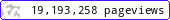•••••MadAsMaths.com :: Project Maths :: Exam Questions

PROJECT MATHS - EXAM QUESTIONS

These presentations contain exam questions on a variety of key mathematical topics.

Note that this is a licensed product if it is to be used for “classroom teaching” so schools or academic institutions must first purchase a licence. Click HERE to contact me.

 algebraic_ordering_gcse_higher.pptangle_calculations.pptarea_perimeter.pptbearings.pptcircle_circumference_and_area.pptcircle_theorems.pptconstructions.pptcylinders_and_cones.pptfraction.pptgraphs_various_topics_gcse_higher.pptinterpreting_miscellaneous_statistical_graphs_and_diagrams.pptirrational_numbers.pptloci.pptnets.pptnumber_problems_worded.pptpercentages.pptpolygons.pptprisms.pptprobability.pptproportionality_formally.pptpythagoras_theorem.pptratio_proportion.pptrounding_and_bounds.pptsequences.pptsimilarity.pptsine_cosine_rule.pptstandard_form.pptsurds.ppttranformations_of_functions_and_graphs.ppttransformation_of_shapes.ppttrigonometry.pptunits_and_mensuration.pptvectors.pptvolume_surface_area.ppt